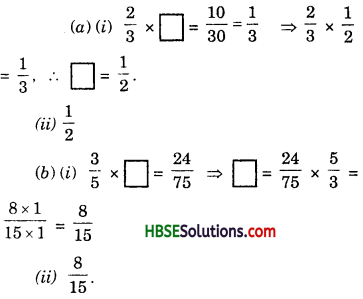# HBSE 7th Class Maths Solutions Chapter 2 Fractions and Decimals Ex 2.3

Haryana State Board HBSE 7th Class Maths Solutions Chapter 2 Fractions and Decimals Ex 2.3 Textbook Exercise Questions and Answers.

## Haryana Board 7th Class Maths Solutions Chapter 2 Fractions and Decimals Exercise 2.3

Question 1.
Find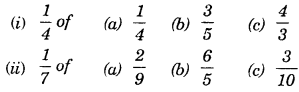Solution: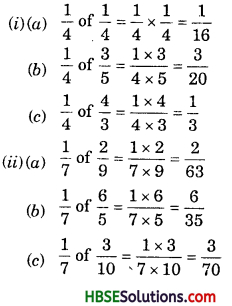Question 2.
Multiply and reduce to lowest form (if possible) :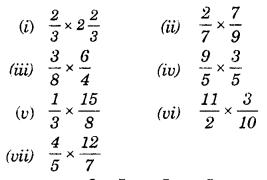Solution: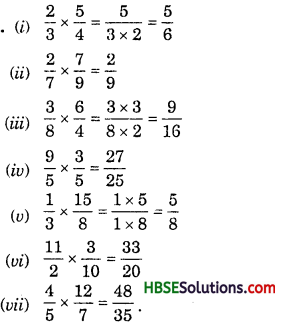Question 3.
Multiply the following fractions :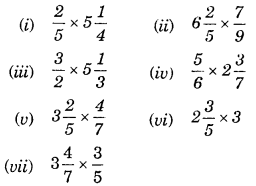Solution: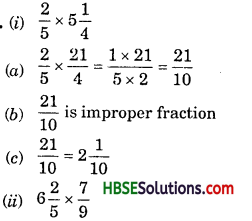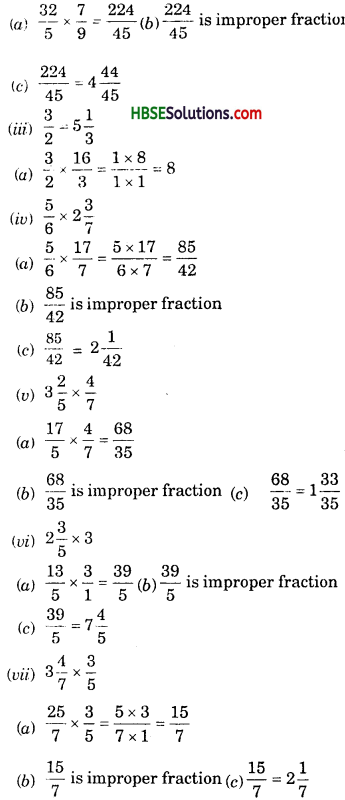Question 4.
Which is greater?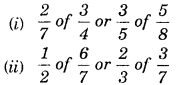Solution: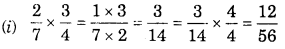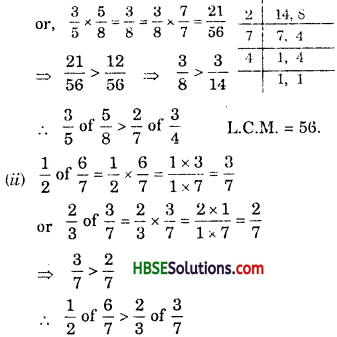Question 5.
Saili plants 4 saplings in a row, in her garden. The distance between two adjacent saplings is $$\frac{3}{4}$$ m. Find the distance between the first and the last sapling.
Solution:
The distance between two adjacent saplings = $$\frac{3}{4}$$ m
There are 4 saplings in a row.
∴ The distance between the first and the last sapling = $$\frac{3}{4}$$ x 3 = $$\frac{9}{4}$$ m.Question 6.
Lipika reads a book for 1 $$\frac{3}{4}$$ hours every day. She reads the entire book in 6 days. IIow many hours in all were required by her to read the book ?
Solution:
Lipika reads a book for $$\frac{7}{4}$$ hours everyday.
∴ Required hours = $$\frac{7}{4} \times 6=\frac{7 \times 3}{2}=\frac{21}{2}=10 \frac{1}{2}$$ hours

Question 7.
A car runs 16 km. using 1 litre of petrol. How much distance will it cover using 2$$\frac{3}{4}$$ litres of petrol ?
Solution:
A car runs 16 km. using 1 litre of petrol.
∴ Total distance cover =16 x 2$$\frac{3}{4}$$
= 16 x $$\frac{11}{4}$$ = 44 km.Question 8.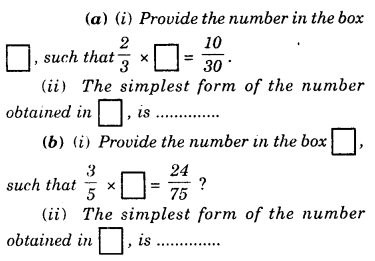Solution: